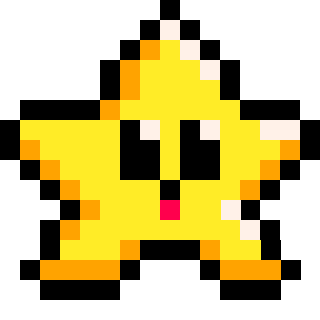# FUNCTION

Imagine that you're working in a big factory that makes all sorts of things. In this factory, there are lots of different machines that perform specific tasks. For example, there might be a machine that cuts metal, another machine that welds pieces together, and a third machine that paints the finished product.

In the same way, when we're writing code, we often need to perform specific tasks over and over again. Instead of copying and pasting the same code every time we need to do that task, we can create a "machine" called a function that does the job for us. Just like the machines in a factory, a function is designed to do one specific thing really well.

Here's an example. Let's say you're writing a program that needs to measure the area of a piece of wood. Instead of writing out the math formula every time you need to use it, you could create a function that performs the calculation for you. The function might look something like this:

``````function measure_wood(width, length)
area = width * length
return area
end``````

In this function, you're telling the computer to calculate the area of a rectangle using the width and length parameters that are passed in. Once the calculation is done, you're passing the result back to wherever the function was told to run.

Just like a machine in a factory, you can use this function over and over again whenever you need to calculate the area of a rectangle. You don't need to remember the math formula or write it out every time - you can just call the function and let it do the work for you.#### Important Terms Related to Functions

###### API

An API is a collection of built-in functions that are pre-made and ready to use. PICO-8 has many pre-made functions such as `print()`, `map()`, and `music()`. You don't have to build your own function for playing music or drawing your map on the screen.

###### Custom Function

A custom function is any function that you create in your game that is not a part of the PICO-8 API.

See create custom function for how and why you would want to make your own.

###### Parameters

A parameter (often shortened to "param") is any data that your function is expecting to receive. You name the parameters in the parentheses when creating your function.

``function name_of_function(param1, param2)``

(commonly also referred to as "arguments")

###### Arguments

An argument (often shortened to "arg") is any data that you pass to your function. You place arguments inside of the parentheses when you call the function to run.

``name_of_function(arg1, arg2)``

("arguments" and "parameters" are often confused when they are used as synonyms but it is important to understand their difference)

###### Call

When you tell a function to run, this is "calling" the function. You call a function simply by writing the function name and including parentheses. You may need to pass data ("arguments") inside of the parentheses when you call the function. If the function is not expecting any data, then empty parentheses are fine.

``name_of_function()``

###### Return

A function can "return" data back to where it was called. In the same way that you can pass data to a function, the function can then pass data back as well. Use the word `return` then the value or variable you wish to pass back. When a value is returned, you must catch it in a variable at the place where the function was called.

``````--create
function measure_perimeter(width, length)
perimeter = width*2 + length*2
return perimeter
end

--call
my_perimeter = measure_perimeter(3,2)

print(my_perimeter) --prints 10``````

It is also possible to return multiple values. Use commas to separate the values you are returning. Also make sure you use commas to catch those values where you call the function.

``````--create
function measure_both(width, length)
area = width * length
perimeter = width*2 + length*2
return area, perimeter
end

--call
my_area, my_perimeter = measure_both(3,2)

print(my_area) --prints 6
print(my_perimeter) --prints 10``````432

4 Nov 2023

`Font`